XGCa
search.F90 File Reference
#include "adios_macro.h"
#include "t_coeff_mod_macro.h"
#include "fftw3.f"
Include dependency graph for search.F90: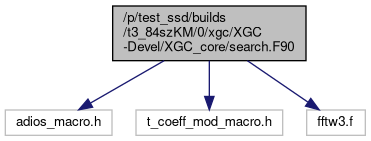## Data Types

module  grid_class

type  grid_class::grid_type

## Functions/Subroutines

subroutine order_nodes (grid, isurf, isize, idx_start, theta, idx)
Sorts input SOL flux-surface according to the poloidal arclength l_theta where l_theta=0 at the outer midplane. More...

subroutine gen_pol_angle_single (i)

subroutine get_b_and_derivs_cyl (r, z, B_mag, br, bphi, bz, dbrdr, dbpdr, dbzdr, dbrdp, dbpdp, dbzdp, dbrdz, dbpdz, dbzdz)

subroutine get_pe_info (mype, totalpe)

subroutine search_tr2 (grid, xy, itr, p)

subroutine search_tr_check_guess (grid, x, init, itr, p)

subroutine convert_001d_2_grid (grid, v1d, v2d)

subroutine convert_001d_2_grid_wrap (v1d, v2d)

subroutine convert_grid_init2 (grid, psn)
Sets up a matrix that evaluates the flux-surface average using only the complete surfaces as specified in an input file generated by the RPI meshing tool. The inverse operation that projects a 1D flux-surface averaged quantity on the 2D mesh is also generated. All vertices that are not enclosed by the complete flux-surfaces (essentially all vertices in the unstructured part of the mesh and in the private flux-region are set to 0! More...

subroutine convert_grid_2_001d (grid, v2d, v1d)

subroutine convert_grid_2_001d_wrap (v2d, v1d)

Initialization of 2nd order accurate 4th derivative radial smoothing operator (hyper-viscosity). On vertices that do not have a well defined radial direction (X-points, mag. axis), an isotropic 2nd derivative hyperviscosity is used as a fallback. More...

subroutine get_node_perp (grid, x, dlperp_in, direction, dist_out, itr, p)

subroutine get_node_theta (grid, x, dltheta_in, direction, dist_out, itr, p)

subroutine get_next_point_gl (x_in, psi_in, dpsi, direction, x_out, dist)

subroutine get_next_point_perp (x_in, dlperp, direction, x_out, dist)

subroutine get_next_point_tang (x_in, dltheta, direction, x_out, dist)

subroutine get_char_length (grid, ip, dl, ierr)

subroutine grid_deriv (grid, qty, qty_deriv_x, qty_deriv_y, psi_only)

subroutine grid_theta_deriv (grid, qty, qty_deriv)

subroutine init_pol_smooth_mat (grid)

subroutine write_pol_smooth_mat

subroutine smooth_pol (grid, qty, qty_smooth)

subroutine smooth_pol_wrap (qty, qty_smooth)

subroutine fourier_filter (grid, filt_inout, inpsi, outpsi, bd_width, op_mode, div_mix)
Interface routine for Fourier filter. More...

subroutine fourier_filter_m_range (grid, mpol_min, mpol_max, n_xdim2, x, x_is_spectral, inpsi, outpsi, bd_width, is_resonant, ntor_real)
Poloidal Fourier filter for the regions 1 and 2 Removes high poloidal mode numbers flux-surfaces while retaining small scale poloidal variations close to the divertor plates. This is achieved by applying a window function that removes the near-divertor part from the data. The windowed data is then low-pass filtered and the high-m variations are removed by calculating x_filtered = (1-win)*x - win*x_filtered. More...

subroutine fourier_filter_update_analytic (x)

subroutine fft_parallel_init (grid)
Initializes the parallelization of the field-aligned FFT filter. Since each flux-surface is filtered separately, the flux-surfaces are distributed among the MPI ranks in the inter-plane communicator (sml_intpl_comm). To achieve approximately even workload, the distribution of flux-surfaces, tries to assign equal numbers of vertices to each rank. More...

subroutine fourier_filter_n_m_range_parallel (grid, nphi, ntor_min, ntor_max, ntor_min_real, ntor_max_real, bands_on, mpol_min, mpol_max, x, op_mode, num_mres_q, inpsi, outpsi, bd_width, div_mix)
Toroidal-poloidal Fourier filter for the regions 1 and 2 This version of the filter is parallelized to a high degree for optimal performance, and allows for multiple toroidal mode numbers. Removes high poloidal mode numbers from flux-surfaces while retaining small scale poloidal variations close to the divertor plates. This is achieved by applying a window function that removes the near-divertor part from the data. The windowed data is then low-pass filtered and the high-m variations are removed by calculating x_filtered = (1-win)*x - win*x_filtered

## NOTE: WINDOW HAS NOT BEEN IMPLEMENTED YET —> USING WIN=1

More...

subroutine fourier_filter_set_nonaligned (grid, x)

subroutine fourier_filter_single_n (grid, filt_inout, inpsi, outpsi, bd_width)
Fourier Filter the input (R,phi,Z data) for a single toroidal mode. More...

subroutine fourier_filter_single_n_flex (grid, ntor, filt_in, filt_out, do_damp, inpsi, outpsi, bd_width)
Fourier Filter the input (R,phi,Z data) for a single toroidal mode that can be different on each calling rank. More...

real(kind=8) function fourier_filter_damp_fac (psi, inpsi, outpsi, bd_width)
Evaluate a damping factor for the boundary condition of the Fourier filter functions. More...

## Function/Subroutine Documentation

 subroutine convert_001d_2_grid ( type(grid_type), intent(in) grid, real (8), dimension(grid%npsi_surf), intent(in) v1d, real (8), dimension(grid%nnode), intent(out) v2d )

Here is the call graph for this function:Here is the caller graph for this function:subroutine convert_001d_2_grid_wrap ( real (8), dimension(grid_global%npsi_surf), intent(in) v1d, real (8), dimension(grid_global%nnode), intent(out) v2d )

Here is the call graph for this function:Here is the caller graph for this function:subroutine convert_grid_2_001d ( type(grid_type), intent(in) grid, real (8), dimension(grid%nnode), intent(in) v2d, real (8), dimension(grid%npsi_surf), intent(out) v1d )

Here is the call graph for this function:Here is the caller graph for this function:subroutine convert_grid_2_001d_wrap ( real (8), dimension(grid_global%nnode), intent(in) v2d, real (8), dimension(grid_global%npsi_surf), intent(out) v1d )

Here is the call graph for this function:Here is the caller graph for this function:subroutine convert_grid_init2 ( type(grid_type), intent(inout) grid, type(psn_type), intent(in) psn )

Sets up a matrix that evaluates the flux-surface average using only the complete surfaces as specified in an input file generated by the RPI meshing tool. The inverse operation that projects a 1D flux-surface averaged quantity on the 2D mesh is also generated. All vertices that are not enclosed by the complete flux-surfaces (essentially all vertices in the unstructured part of the mesh and in the private flux-region are set to 0!

Parameters
 grid[inout] type(grid_type), grid information psn[in] field data (esp. wall nodes), type(psn_type)

Here is the call graph for this function:Here is the caller graph for this function:subroutine fft_parallel_init ( type(grid_type), intent(inout) grid )

Initializes the parallelization of the field-aligned FFT filter. Since each flux-surface is filtered separately, the flux-surfaces are distributed among the MPI ranks in the inter-plane communicator (sml_intpl_comm). To achieve approximately even workload, the distribution of flux-surfaces, tries to assign equal numbers of vertices to each rank.

Parameters
 [in,out] grid grid data structure, type(grid_type)

Here is the call graph for this function:Here is the caller graph for this function:subroutine fourier_filter ( type(grid_type), intent(in) grid, real (kind=8), dimension(grid%nnode), intent(inout) filt_inout, real (8), intent(in) inpsi, real (8), intent(in) outpsi, real (8), intent(in) bd_width, integer, intent(in) op_mode, logical, intent(in) div_mix )

Interface routine for Fourier filter.

Parameters
 [in] grid grid data, type(grid_type) [in,out] filt_inout input real part, real(8) [in] inpsi Inner filter boundary, real(8) [in] outpsi Outer filter boundary, real(8) [in] bd_width Boundary width, real(8) [in] op_mode (1) Simple single-n toroidal mode filter (4) Simple poloidal mode filter with m-range (2,5) n-m_range with m=nq+/-mres (parallel version 2, operational, includes side bands) (3,6) simple n-m range (parallel version 2, operational) Former modes (2) and (3) are deprecated and have been merged with (5) and (6) [in] div_mix Whether to blend unfiltered data near divertor with filtered data due to windowing function in SOL, logical

Here is the call graph for this function:Here is the caller graph for this function:real (kind=8) function fourier_filter_damp_fac ( real (kind=8), intent(in) psi, real (kind=8), intent(in) inpsi, real (kind=8), intent(in) outpsi, real (kind=8), intent(in) bd_width )

Evaluate a damping factor for the boundary condition of the Fourier filter functions.

Parameters
 [in] psi Input poloidal flux, real(8) [in] inpsi Inner filter boundary, real(8) [in] outpsi Outer filter boundary, real(8) [in] bd_width Boundary width, real(8)

Here is the caller graph for this function: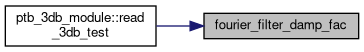subroutine fourier_filter_m_range ( type(grid_type), intent(in) grid, integer, intent(in) mpol_min, integer, intent(in) mpol_max, integer, intent(in) n_xdim2, real (kind=8), dimension(grid%nnode,n_xdim2), intent(inout) x, logical, intent(in) x_is_spectral, real (kind=8), intent(in) inpsi, real (kind=8), intent(in) outpsi, real (kind=8), intent(in) bd_width, logical, intent(in) is_resonant, integer, intent(in) ntor_real )

Poloidal Fourier filter for the regions 1 and 2 Removes high poloidal mode numbers flux-surfaces while retaining small scale poloidal variations close to the divertor plates. This is achieved by applying a window function that removes the near-divertor part from the data. The windowed data is then low-pass filtered and the high-m variations are removed by calculating x_filtered = (1-win)*x - win*x_filtered.

Extension: Input x can now have shape (:,:) with the size of the 2nd dimension given by the input parameter n_xdim2 (batched filtering). If n_xdim2=2 and x_is_spectral=.true., then the inputs are the cosine (1) and sine (2) components of a discrete toroidal Fourier transform in sin/cos representation. In this case a transformation is applied after the poloidal FFT so that only the poloidal mode numbers around the resonance $$m=n*q$$ are retained, and the rest is discarded. Assume $$f = [a*\cos(n*\phi-m*\theta)+b*\sin(n*\phi-m*\theta)]+[c*\cos(n*\phi+m*\theta)+d*\sin(n*\phi+m*\theta)]$$ with m>0. Whether $$+m$$ or $$-m$$ is required depends on the orientation of the magnetic field, and to which band of the $$(n,m)$$ spectrum the mode belongs. The sign of $$m$$ is given by $$\sigma = \mathtt{sml_bt_sign}\cdot\mathtt{sml_bp_sign}\cdot(-1)^{i}$$, with $$i=[(\mathtt{ntor_real}/\mathtt{sml_wedge_n})-1]\cdot(2/\mathtt{sml_nphi_total})$$ is the side-band index of the toroidal mode number under consideration.

Let f_cnm and f_snm be the complex-valued Fourier coefficients resulting from the FFT of the cosine and sine components of the toroidal DFT for the numerical toroidal mode number n.

Then we have: Re(f_cnm) = a+c Im(f_cnm) = b-d Re(f_snm) = b+d Im(f_snm) =-a+c

Hence: (f_cnm + I*f_snm)/2 = (a+I*b) (f_snm + I*f_cnm)/2 = (d+I*c)

So if $$\sigma=+1$$, we need the coefficients a and b, and use f_cnm <- Re[(f_cnm + I*f_snm)/2] + I*Im[(f_cnm + I*f_snm)/2] f_snm <- Im[(f_cnm + I*f_snm)/2] - I*Re[(f_cnm + I*f_snm)/2]

and if $$\sigma=-1$$, we need c and d, and use f_cnm <- Im[(f_snm + I*f_cnm)/2] - I*Re[(f_snm + I*f_cnm)/2] f_snm <- Re[(f_snm + I*f_cnm)/2] + I*Im[(f_snm + I*f_cnm)/2]

Parameters
 [in] grid grid data, type(grid_type) [in] mpol_min minimal poloidal mode number, integer [in] mpol_max maximal poloidal mode number, integer [in,out] x input data, shape either (gridnnode) or (gridnnode,:), real(8) [in] n_xdim2 Size of second dimension; 1 if shape(x) is (:), integer [in] x_is_spectral If true, x(:,:) are the cosine and sine coefficients of a discrete toroidal DFT, logical [in] inpsi Inner filter boundary, real(8) [in] outpsi Outer filter boundary, real(8) [in] bd_width Boundary width, real(8) [in] is_resonant If .true. input m-range is ignored and instead set according to |m/q(psi)-ntor_real|<=sml_mode_select_mres_q, logical [in] ntor_real (Real) toroidal mode number used for setting the filter range

Here is the call graph for this function:Here is the caller graph for this function:subroutine fourier_filter_n_m_range_parallel ( type(grid_type), intent(in) grid, integer, intent(in) nphi, integer, intent(in) ntor_min, integer, intent(in) ntor_max, integer, intent(in) ntor_min_real, integer, intent(in) ntor_max_real, logical, intent(in) bands_on, integer, intent(in) mpol_min, integer, intent(in) mpol_max, real (kind=8), dimension(grid%nnode), intent(inout) x, integer, intent(in) op_mode, integer, intent(in) num_mres_q, real (kind=8), intent(in) inpsi, real (kind=8), intent(in) outpsi, real (kind=8), intent(in) bd_width, logical, intent(in) div_mix )

Toroidal-poloidal Fourier filter for the regions 1 and 2 This version of the filter is parallelized to a high degree for optimal performance, and allows for multiple toroidal mode numbers. Removes high poloidal mode numbers from flux-surfaces while retaining small scale poloidal variations close to the divertor plates. This is achieved by applying a window function that removes the near-divertor part from the data. The windowed data is then low-pass filtered and the high-m variations are removed by calculating x_filtered = (1-win)*x - win*x_filtered

## NOTE: WINDOW HAS NOT BEEN IMPLEMENTED YET —> USING WIN=1

Parameters
 [in] grid grid data, type(grid_type) [in] nphi number of toroidal grid points, integer [in] ntor_min minimal numerical toroidal mode number, integer [in] ntor_max maximal numerical toroidal mode number, integer [in] ntor_min_real minimal real (incl. wedge factor) toroidal mode number, integer [in] ntor_max_real maximal real (incl. wedge factor) toroidal mode number, integer [in] bands_on Whether to use only the main band of resonant modes or also side bands (n>sml_nphi_total/2), logical [in] mpol_min minimal poloidal mode number, integer [in] mpol_max maximal poloidal mode number, integer [in,out] x input data, real(8) [in] op_mode mode of operation (integer): 1) simple range of pol. mode numbers, 2) resonant pol. modes [in] num_mres_q number of poloidal modes retained around the res. mode divided by the safety factor in op_mode=2 [in] inpsi Inner filter boundary, real(8) [in] outpsi Outer filter boundary, real(8) [in] bd_width Boundary width, real(8) [in] div_mix Whether to blend unfiltered data near the divertor with filtered data (due to windowing function), logical

Here is the call graph for this function:Here is the caller graph for this function:subroutine fourier_filter_set_nonaligned ( type(grid_type), intent(in) grid, real (kind=8), dimension(grid%nnode), intent(inout) x )

Here is the call graph for this function:Here is the caller graph for this function:subroutine fourier_filter_single_n ( type(grid_type), intent(in) grid, real (kind=8), dimension(grid%nnode), intent(inout) filt_inout, real (kind=8), intent(in) inpsi, real (kind=8), intent(in) outpsi, real (kind=8), intent(in) bd_width )

Fourier Filter the input (R,phi,Z data) for a single toroidal mode.

Parameters
 [in] grid grid data, type(grid_type) [in,out] filt_inout input real part, real(8) [in] inpsi Inner filter boundary, real(8) [in] outpsi Outer filter boundary, real(8) [in] bd_width Boundary width, real(8)

Here is the call graph for this function:Here is the caller graph for this function:subroutine fourier_filter_single_n_flex ( type(grid_type), intent(in) grid, integer, intent(in) ntor, real (kind=8), dimension(grid%nnode), intent(in) filt_in, real (kind=8), dimension(grid%nnode,2), intent(out) filt_out, logical, intent(in) do_damp, real (kind=8), intent(in) inpsi, real (kind=8), intent(in) outpsi, real (kind=8), intent(in) bd_width )

Fourier Filter the input (R,phi,Z data) for a single toroidal mode that can be different on each calling rank.

Parameters
 [in] grid grid data, type(grid_type) [in] ntor Toroidal mode number, integer [in] filt_in input, real(8) [out] filt_out output sin and cos components, real(8) [in] do_damp Whether to use a psi-window function, logical [in] inpsi Inner filter boundary, real(8) [in] outpsi Outer filter boundary, real(8) [in] bd_width Boundary width, real(8)

Here is the call graph for this function:Here is the caller graph for this function:subroutine fourier_filter_update_analytic ( real (kind=8), dimension(grid_global%nnode), intent(inout) x )

Here is the call graph for this function:Here is the caller graph for this function:subroutine calc_gen_theta_psi::gen_pol_angle_single ( integer, intent(in) i )

Here is the call graph for this function: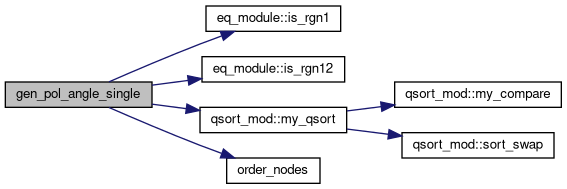Here is the caller graph for this function: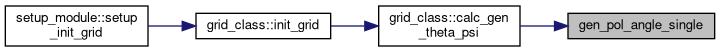subroutine get_b_and_derivs_cyl ( real(kind=8), intent(in) r, real(kind=8), intent(in) z, real(kind=8), intent(out) B_mag, real(kind=8), intent(out) br, real(kind=8), intent(out) bphi, real(kind=8), intent(out) bz, real(kind=8), intent(out) dbrdr, real(kind=8), intent(out) dbpdr, real(kind=8), intent(out) dbzdr, real(kind=8), intent(out) dbrdp, real(kind=8), intent(out) dbpdp, real(kind=8), intent(out) dbzdp, real(kind=8), intent(out) dbrdz, real(kind=8), intent(out) dbpdz, real(kind=8), intent(out) dbzdz )

Here is the call graph for this function: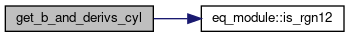Here is the caller graph for this function: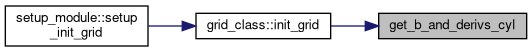subroutine get_char_length ( type (grid_type), intent(inout) grid, integer, intent(in) ip, real (kind=8), dimension(3), intent(out) dl, integer, intent(out) ierr )

Here is the call graph for this function:Here is the caller graph for this function:subroutine get_next_point_gl ( real (kind=8), dimension(2), intent(in) x_in, real (kind=8), intent(in) psi_in, real (kind=8), intent(in) dpsi, integer, intent(in) direction, real (kind=8), dimension(2), intent(inout) x_out, real (kind=8), intent(inout) dist )

Here is the caller graph for this function:subroutine get_next_point_perp ( real (kind=8), dimension(2), intent(in) x_in, real (kind=8), intent(in) dlperp, integer, intent(in) direction, real (kind=8), dimension(2), intent(inout) x_out, real (kind=8), intent(inout) dist )

Here is the caller graph for this function:subroutine get_next_point_tang ( real (kind=8), dimension(2), intent(in) x_in, real (kind=8), intent(in) dltheta, integer, intent(in) direction, real (kind=8), dimension(2), intent(inout) x_out, real (kind=8), intent(inout) dist )

Here is the call graph for this function:Here is the caller graph for this function:subroutine get_node_perp ( type (grid_type), intent(in) grid, real (kind=8), dimension(2), intent(in) x, real (kind=8), intent(in) dlperp_in, integer, intent(in) direction, real (kind=8), intent(inout) dist_out, integer, intent(inout) itr, real (kind=8), dimension(3), intent(inout) p )

Here is the call graph for this function:Here is the caller graph for this function:subroutine get_node_theta ( type (grid_type), intent(in) grid, real (kind=8), dimension(2), intent(in) x, real (kind=8), intent(in) dltheta_in, integer, intent(in) direction, real (kind=8), intent(inout) dist_out, integer, intent(inout) itr, real (kind=8), dimension(3), intent(inout) p )

Here is the call graph for this function:Here is the caller graph for this function:subroutine get_pe_info ( integer mype, integer totalpe )
 subroutine grid_deriv ( type(grid_type), intent(in) grid, real (kind=8), dimension(grid%nnode), intent(in) qty, real (kind=8), dimension(grid%nnode), intent(inout) qty_deriv_x, real (kind=8), dimension(grid%nnode), intent(inout) qty_deriv_y, logical, intent(in) psi_only )

Here is the call graph for this function:Here is the caller graph for this function:subroutine grid_theta_deriv ( type(grid_type), intent(in) grid, real (kind=8), dimension(grid%nnode), intent(in) qty, real (kind=8), dimension(grid%nnode), intent(inout) qty_deriv )

Here is the call graph for this function:subroutine init_pol_smooth_mat ( type (grid_type), intent(inout) grid )

Here is the call graph for this function:Here is the caller graph for this function:subroutine init_rad_smooth ( type (grid_type), intent(inout) grid )

Initialization of 2nd order accurate 4th derivative radial smoothing operator (hyper-viscosity). On vertices that do not have a well defined radial direction (X-points, mag. axis), an isotropic 2nd derivative hyperviscosity is used as a fallback.

Parameters
 [in,out] grid XGC grid data structure, type(grid_type)

Here is the call graph for this function:Here is the caller graph for this function:subroutine make_v_dot_grad_mat ( type(grid_type), intent(inout) grid, type(mat_type), intent(out) mat, real (kind=8), dimension(grid%nnode,3), intent(in) v )

Here is the call graph for this function:subroutine calc_gen_theta_psi::order_nodes ( type(grid_type), intent(in) grid, integer, intent(in) isurf, integer, intent(in) isize, integer, intent(in) idx_start, real (kind=8), dimension(isize), intent(out) theta, integer, dimension(isize), intent(out) idx )

Sorts input SOL flux-surface according to the poloidal arclength l_theta where l_theta=0 at the outer midplane.

Parameters
 [in] grid grid data; type(grid_type) [in] isurf flux-surface index; integer [in] isize length of the flux-surface; integer [out] theta output arclength; real(8) [out] idx output sort index; integer

Here is the caller graph for this function: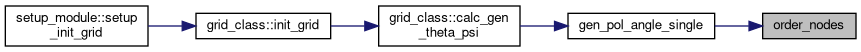subroutine search_tr2 ( type(grid_type) grid, real(kind=8), dimension(2) xy, integer itr, real(kind=8), dimension(3) p )

Here is the caller graph for this function: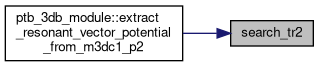subroutine search_tr_check_guess ( type(grid_type), intent(in) grid, real (kind=8), dimension(2), intent(in) x, integer, intent(in) init, integer, intent(out) itr, real (kind=8), dimension(3), intent(out) p )

Here is the call graph for this function: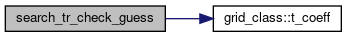Here is the caller graph for this function:subroutine smooth_pol ( type(grid_type), intent(in) grid, real (kind=8), dimension(grid%nnode), intent(in) qty, real (kind=8), dimension(grid%nnode), intent(inout) qty_smooth )

Here is the call graph for this function:Here is the caller graph for this function:subroutine smooth_pol_wrap ( real (kind=8), dimension(grid_global%nnode), intent(in) qty, real (kind=8), dimension(grid_global%nnode), intent(inout) qty_smooth )

Here is the call graph for this function:Here is the caller graph for this function:subroutine write_gradient_mat ( type (grid_type), intent(inout) grid )

Here is the call graph for this function: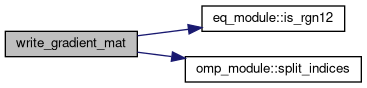Here is the caller graph for this function: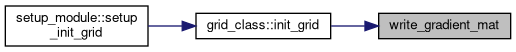subroutine init_pol_smooth_mat::write_pol_smooth_mat ( )

Here is the caller graph for this function: